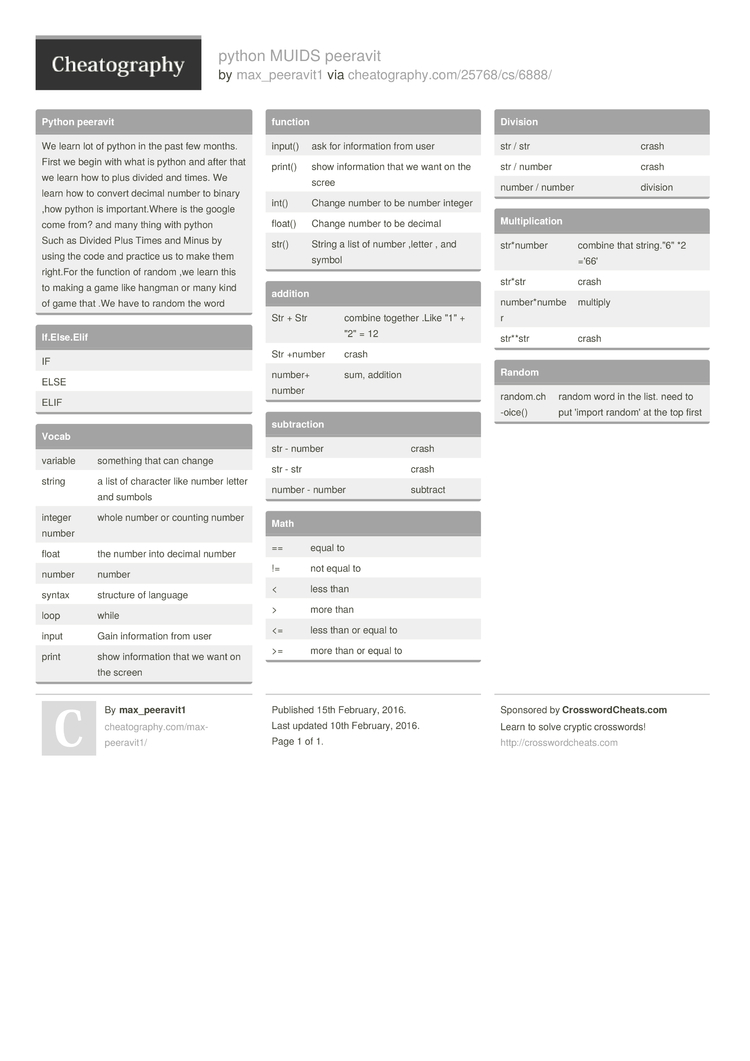# python MUIDS peeravit by max_peeravit1

### Python peeravit

 We learn lot of python in the past few months. First we begin with what is python and after that we learn how to plus divided and times. We learn how to convert decimal number to binary ,how python is import­ant.Where is the google come from? and many thing with python Such as Divided Plus Times and Minus by using the code and practice us to make them right.For the function of random ,we learn this to making a game like hangman or many kind of game that .We have to random the word

### If.Els­e.Elif

 IF ELSE ELIF

### Vocab

 variable something that can change string a list of character like number letter and sumbols integer number whole number or counting number float the number into decimal number number number syntax structure of language loop while input Gain inform­ation from user print show inform­ation that we want on the screen

### function

 input() ask for inform­ation from user print() show inform­ation that we want on the scree int() Change number to be number integer float() Change number to be decimal str() String a list of number ,letter , and symbol

 Str + Str combine together .Like "­1" + "­2" = 12 Str +number crash number+ number sum, addition

### subtra­ction

 str - number crash str - str crash number - number subtract

### Math

 == equal to != not equal to < less than > more than <= less than or equal to >= more than or equal to

### Division

 str / str crash str / number crash number / number division

### Multip­lic­ation

 str*number combine that string."6" *2 ='66' str*str crash number­*number multiply str**str crash

### Random

 random.ch­-oice() random word in the list. need to put 'import random' at the top first1 Page
//media.cheatography.com/storage/thumb/max-peeravit1_python-muids-peeravit.750.jpg

PDF (recommended)